# Quiz 2: Accounting and Financial Decision Making

Business

The financial statements of a company represent the information regarding the financial performance of the company for a period of time and its financial position on a particular date to its users. These users like investors, creditors, suppliers and shareholders of financial information evaluate these financial statements in mainly three areas which are profitability, liquidity and solvency. Financial ratio: It refers to the technique of depicting the relationship between two predetermined numerical values. For instance current ratio depicts the relationship between current assets and current liabilities. Balance sheet: It is the financial statement which includes the assets, liabilities and equity of business at a particular time. It identifies the financial position of company.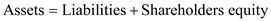a. Current Assets are the valuables that can be converted into or exchanged in cash or cash equivalent. The time span of the conversion of the asset into cash is one year. It is required to compute the value of current assets which can be calculated by adding the values of cash, accounts receivables (Net), inventory, prepaid expenses and marketable securities. These are all current assets because they can be easily converted to cash by selling them in the market within a period of one year or less.Hence, current assets are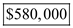. Current Liabilities are the payment or credit that is due or is supposed to be paid in near future. The value of current liabilities can be computed by adding the values of accounts payable, notes payable and accrued expenses because they have to be paid within one year or less. These values are taken from the income statement of the company to compute the current liabilities as:Hence, current liabilities are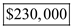. Working capital is the money that is to be used in the day in or day out of the basic business. The difference between the current assets and the current liabilities is the working capital. It is computed as shown below:Hence, working capital is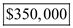. Shareholder's equity is the net worth availability of the owner the money left with the owners after all the expenses in the form of debts.Hence, shareholder's equity is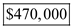. b. Earnings per share (EPS): It indicates the portion of earnings of the company which is allocated to company's each outstanding common stock. It is the ratio which is determined by dividing net income excluding dividend of preference by number of outstanding shares. Computation of EPS for 2018 (the number of shares and net income in millions) is given below:Hence, the EPS of company is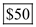. c. Par value: It means the sale price of the share at the initial stage of the company. The company can sell the shares at par value or above or below the par value which depends upon the company's market value. The information about the treasury stock can be analyzed from the balance sheet, the column above the stockholders; equity. Hence, it can be said that the par value of the each share common stock is \$15. The capital surplus is given as \$150,000. The market value of the stock at the issue of the common shares can be computed as shown below:Hence, market value per share is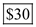.

There is no answer for this question

a) First, find the working-capital requirement for the chemical company. In order to do this properly, one would need to have the net value for the current assets and current liabilities that the company has at some point in time in order to get this amount. This will be shown in the below table.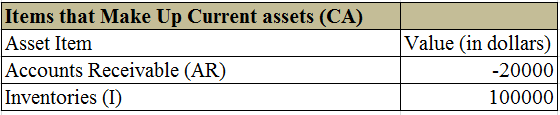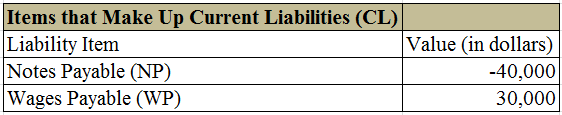From the above information, take the difference between the assets and liabilities to find the working capital requirement for the first project year. Use the following formula to calculate the working-capital requirement: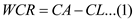Here,Calculations:Thus, the amount of working capital ratio for the chemical company after the first year is approximately \$90,000.00. Now, find the taxable income. Use the following formula to calculate the taxable income: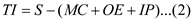Here,Here, MC includes the values for direct materials, direct labor, overhead, and depreciation.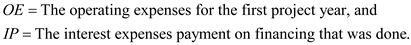Calculations:Thus, the amount of taxable income that is applicable to the chemical plant and their pursuits to wanting to add a second facility to their location is approximately \$680,000.00. c) Use the following formula to find out the net income: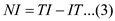Here,Calculations:Thus, the amount of net income that is applicable to the chemical plant and their pursuits to wanting to add a second facility to their location is approximately \$408,000.00. d) Now, determine the net cash flow from operating activities. Use the following formula to calculate the net cash flow from operating activities: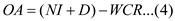Here,Calculations:Thus, the net cash flow for the firm from activities for the first project year is \$518,000. Now, determine the net cash flow for the company. Here, one must take into account the investing and financing activities that the chemical plant did over the first project year, because these will either benefit or hurt the net cash flow. Use the following formula to calculate the net cash flow: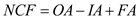Here,Calculations:Thus, the net cash flow is \$318,000.00.

There is no answer for this question

There is no answer for this question